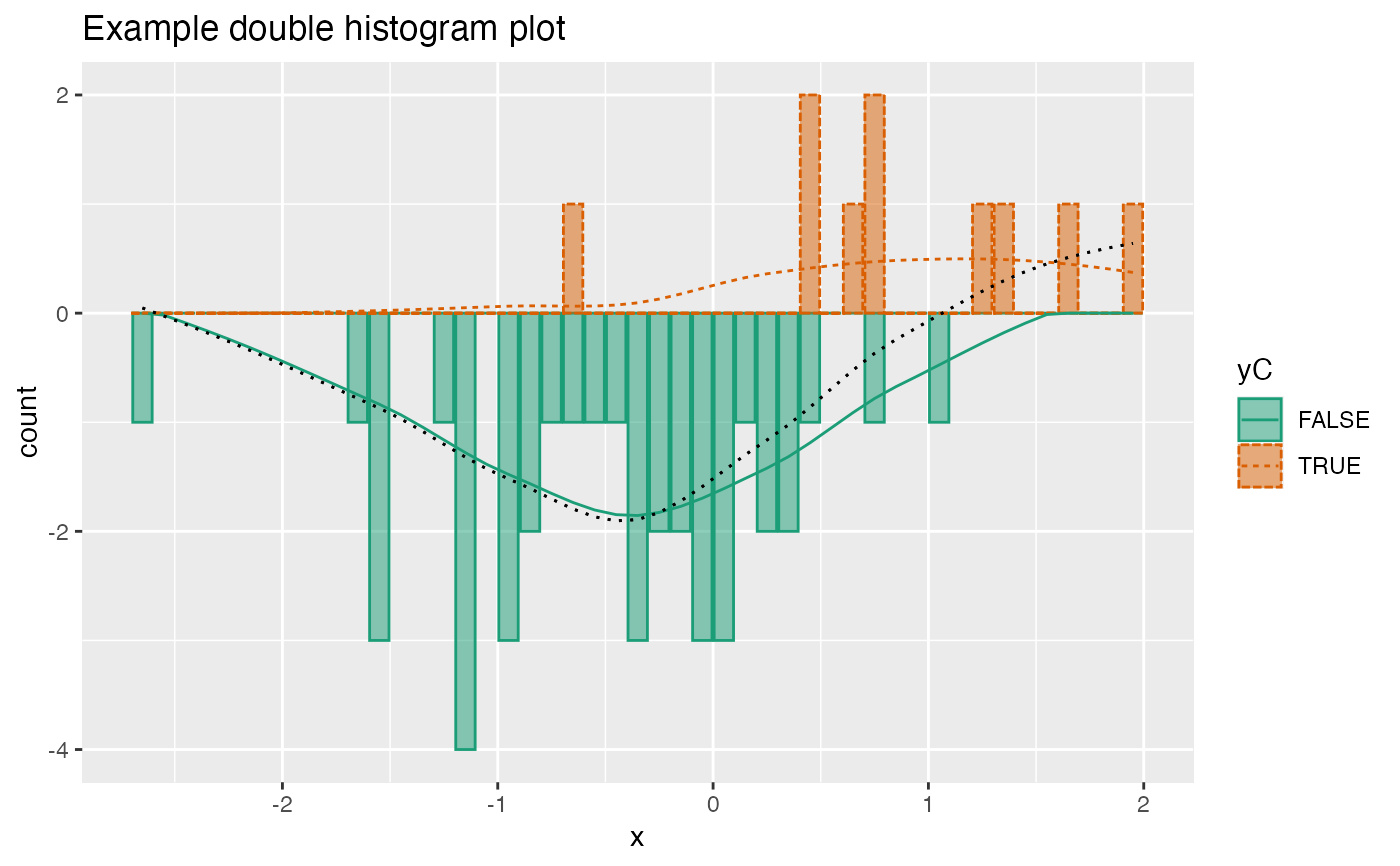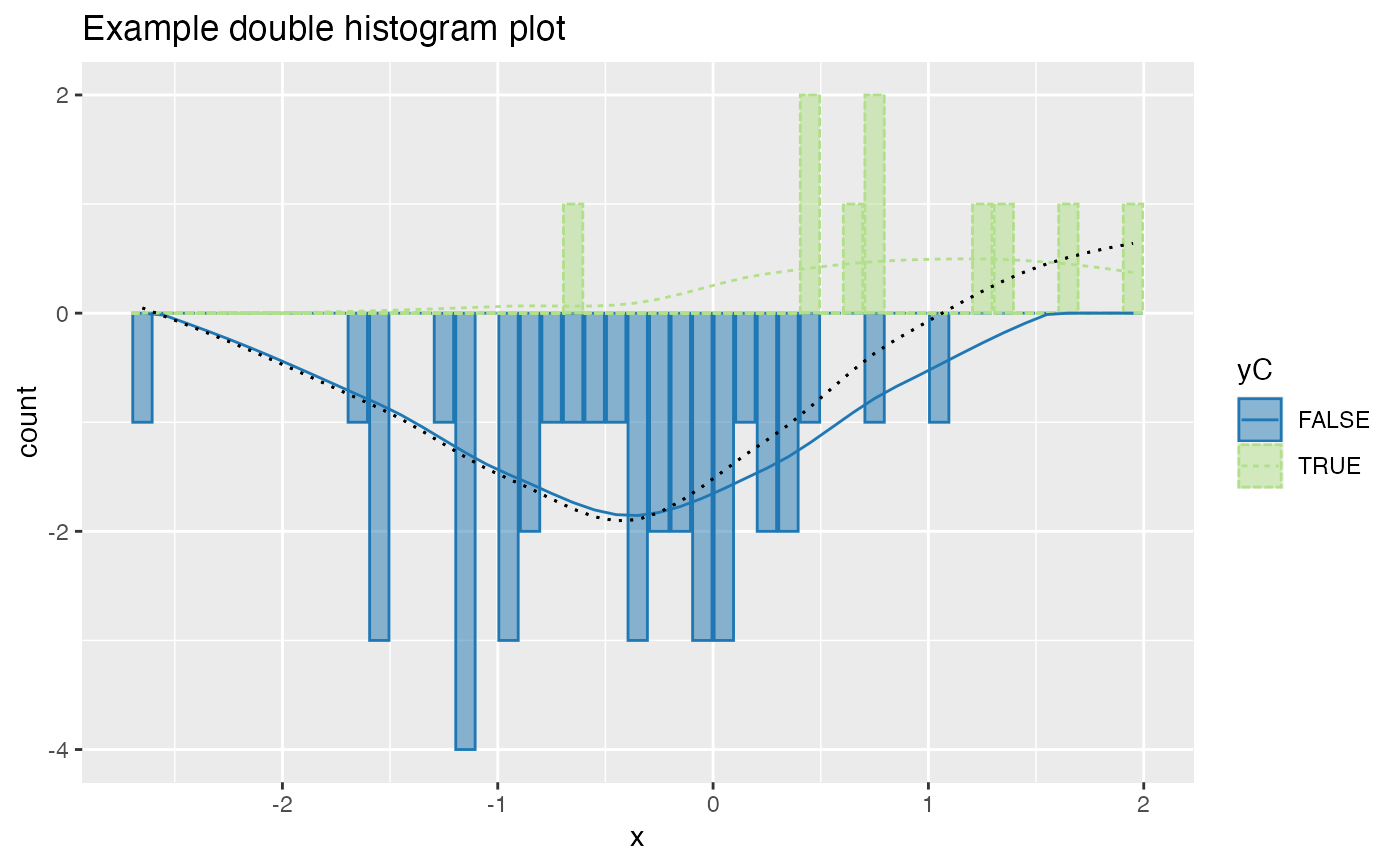Plot two histograms conditioned on a binary outcome variable.

DoubleHistogramPlot(
frame,
xvar,
truthVar,
title,
...,
palette = "Dark2",
breaks = 40
)

## Arguments

frame data frame to get values from name of the independent (input or model) column in frame name of the dependent (output or result to be modeled) column in frame title to place on plot no unnamed argument, added to force named binding of later arguments. name of Brewer palette (can be NULL) breaks to pass to histogram

## Details

To distinguish the two conditions, one histogram is plotted upside-down.

The use case for this visualization is to plot a predictive model score (usually the predicted probability of a desired outcome) conditioned on the actual outcome. However, you can use it to compare any numerical quantity conditioned on a binary feature.

If palette is NULL, plot colors will be chosen from the default ggplot2 palette. Setting palette to NULL allows the user to choose a non-Brewer palette, for example with scale_fill_manual.

## Examples


set.seed(34903490)
x = rnorm(50)
y = 0.5*x^2 + 2*x + rnorm(length(x))
frm = data.frame(x=x,y=y,yC=y>=as.numeric(quantile(y,probs=0.8)))
frm$absY <- abs(frm$y)
frm$posY = frm$y > 0
frm\$costX = 1
WVPlots::DoubleHistogramPlot(frm, "x", "yC", title="Example double histogram plot")# redo the plot with a custom palette
plt = WVPlots::DoubleHistogramPlot(frm, "x", "yC", palette=NULL,
title="Example double histogram plot")
cmap = c("TRUE" = "#b2df8a", "FALSE" = "#1f78b4")
plt + ggplot2::scale_color_manual(values=cmap) +
ggplot2::scale_fill_manual(values=cmap)